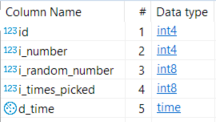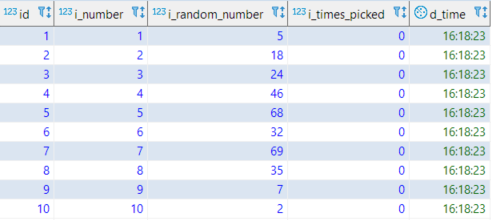# Use of Floor Function in Postgres SQL

Get Started >>

## Introduction

In this tutorial, we learn to use the Floor function in our Postgres SQL queries. We’ll begin easy and then add some complexity in order to best understand the scope and use of this function. Here we will explore:

• What? From the highest level, what does the FLOOR function do?
• Why? What are a couple situations where we would use the Floor() function?
• How? How do we use this function in our PostgreSQL database queries?
• More We’ll also learn about the Random and Generate_Series functions.

## Prerequisites

• Understanding of writing SQL for Postgres (or any other relational database, like Oracle, MS SQL Server, MySQL, etc.) using the free PostgreSQL Admin tool or some other RDB administration app, or via code designed to read and write to databases, including Python, PHP, Java, Javascript, C#, ASP.Net, VB.Net, Ruby, Node, etc.
• Knowledge of some basic SQL statements, like `SELECT`, `FROM`, `INSERT`, `UPDATE`, and `GROUP BY`.

## What is the FLOOR() function and how does it work?

The Postgres `FLOOR()` function returns the highest value integer that is equal to or smaller than the supplied number. In other words, it chops off the decimal point.

Syntax

### FLOOR()

• Input: 1.23552135, Output: 1
• Input: 4.90257826, Output: 4
• Input: 2.53824445, Output: 2
• Input: -2.73824445, Output: -3
• Input: -2.10824445, Output: -3

Did you notice anything strange from the above examples? Look again at the last two. Because of the other examples, we might think our output would be 2. However, when we remember the following rule about how Floor works, we’ll see why our output for both should be -3:

“… returns the highest value integer that is `equal to or smaller` than the supplied number…”

Note: It’s like the INT() function in many programming languages.

## Why and when would we use the FLOOR() function?

One way we may need to use the Floor function is when generating random numbers because Postgres’ RANDOM function returns numbers that are many precision points below the decimal point. So we’ll begin there.

First, let’s take a quick look at how the FLOOR() and RANDOM() function work together.

 12345 FLOOR(RANDOM()*10) FLOOR(RANDOM()*10)+1 FLOOR(RANDOM()*(i_largest-i_smallest)+i_smallest)

### Analysis

• `FLOOR(RANDOM()*10)`: returns a random between (and including) 0 and 9.
• `FLOOR(RANDOM()*10)+1`: returns a random between (and including) 1 and 10.
• `FLOOR(RANDOM()*(i_largest-i_smallest)+i_smallest)`: returns a random integer between (and including) i_smallest and i_largest.

## Now let’s begin building a mini-project

### Our target goal

We want to fill a table with random numbers. We’ll begin by creating a practice table called “test_random”:

 12345678 CREATE TABLE public.test_random (     id serial NOT NULL     , i_number int4 NULL DEFAULT 0     , i_random_number int8 NULL DEFAULT 0     , i_times_picked int8 NOT NULL DEFAULT 0     , d_time TIME NULL DEFAULT now() ); CREATE UNIQUE INDEX test_random_id_idx ON public.test_random USING btree (id);

The SQL above should give you a table like this:

[](https://gyazo.com/19d647e80894097aba5de18ee2c125ab “Floor function Postgres SQL”)

### Analysis of the table we just created

• `id`: This is just good database design practice, to create a table with an indexed id field. Quite often you will need a field like this with a unique index, especially in relational databases where you want to normalize. Also, we set ours to auto-increment, which is another common practice we recommend.
• `i_number`: This column is purely so we can easily follow what is going on when we insert and update data in our test_random table.
• `i_random_number`: This is the bread & butter of our project. This is the column where we store the random numbers we create using the Postgres RANDOM() function.
• `i_times_picked`: This is the field we will use to store our calculation later when we UPDATE the table with a count (using the COUNT() function) of how many times any given random number popped up. We’ll use that in order to double-check just how random our numbers are.
• `d_time`: This is another column we put in for your future use of this table, in case you decide to do testing to find out speeds of various techniques of using the RANDOM function in your SQL queries. Here, we are working with small datasets, but you could choose to change the “generate_series()” function to generate hundreds of thousands of rows instead of the small number we do here in a minute. And if you do that, you may wish to compare how much time it takes to run.

### Step 2. Fill our new table with data

 1234567891011 INSERT INTO     test_random     (     i_number     , i_random_number     ) SELECT     i AS i_number     , FLOOR(RANDOM()*100) FROM     generate_Series(1,10) AS i;

### SQL Analysis

• (1) `INSERT INTO`: This is where we tell PostgreSQL that we are going to insert data into two columns (fields) and we named those fields “i_number” and “i_random_number”.
• (2) `SELECT`: Generate the data to go in the two columns we mentioned above. We’re getting “i” later in our FROM section. The next line, `FLOOR(RANDOM()*100)` is what gives us a random integer between 0 and 100.
• (3) `FROM`: Uses the `generate_Series(i_start, i_end)` function to generate 10 numbers in sequence, like so: 1, 2, 3, 4, 5, 6, 7 8, 9, 10. This will also cause our FROM to generate 10 rows, new rows because of the INSERT statement at the top.

After executing the SQL above, your `test_random` table should have 10 rows and look just like this, except with different numbers in the “i_random_number” column:

[](https://gyazo.com/b861f1c6b4a1f08ee3f05edd37ad9630 “Table after insert of Random integers”)

OK. Yay, the numbers in our `i_random_number` column look to be random!

Now, we need to keep in mind we have generated only 10 numbers and really, that’s not enough to know anything about how random our distribution is. Also, we don’t have any duplicates, so no need to utilize our `i_times_picked` column yet. So let’s generate more rows using the same query as before, except changing the line in our SQL that says `generate_Series(1,10) AS i;` to use “20” instead of “10”.

### Step 3: Add 20 more rows to our 10-row dataset.

 1234567891011 INSERT INTO     test_random     (     i_number     , i_random_number     ) SELECT     i AS i_number     , FLOOR(RANDOM()*100) FROM     generate_Series(1,20) AS i;

Now our data set has 30 records with random numbers. Take a look at your dataset. Are there any duplicate numbers in the `i_random_number` column? If not, run that INSERT query again. Keep running it until your resulting data set has at least one duplicate in the `i_random_number` field. If you are curious, you can set your “generate_series” function to create a few thousand rows. The more you create, the more likely we’ll be to find any patterns that may exist

## Conclusion

In this lesson, we learned how to use PostgreSQL’s `FLOOR()` function to turn numbers into integers in SQL statements. We also used a real world example. We also worked with other functions, including `RANDOM()`, `GENERATE_SERIES()`, and `COUNT()`.

Rate## Pilot the ObjectRocket Platform Free!

Try Fully-Managed CockroachDB, Elasticsearch, MongoDB, PostgreSQL (Beta) or Redis.

## Keep in the know!

Subscribe to our emails and we’ll let you know what’s going on at ObjectRocket. We hate spam and make it easy to unsubscribe.